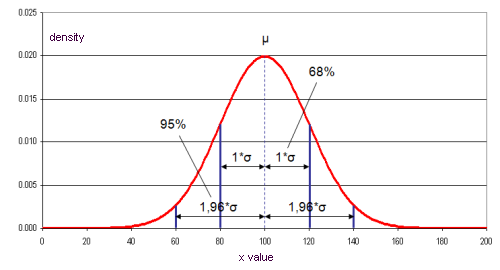STAN allows the consideration of data uncertainties.

It is assumed that uncertain quantities are normally distributed, given by their mean value and standard deviation. This approximation offers the possibility to use methods like error propagation and data reconciliation.

e.g. normally distributed random quantity with mean value 100 and standard deviation 2095 percent of all data can be found within the interval mean value +/- two times the standard deviation. Because of that it is called a 95% confidence interval.

While the standard deviation (s) describes the deviation of the sample the standard uncertainty (u) describes the deviation of the mean value. The standard uncertainty is calculated by dividing the standard deviation by the square root of the sample number n.

In STAN the standard uncertainty should be used.